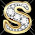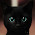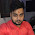## Wednesday, 19 December 2012

### FIFO(First In First Out) Buffer in Verilog

A FIFO(First in First Out) buffer is an elastic storage usually used between two subsystems. As the name indicates the memory that is first written into the FIFO is the first to be read or processed. A FIFO has two control signals i.e. write and read. When write is enabled data is written into the buffer and when read is enabled data is "removed" from the buffer to make room for more data. This concept of write and read (remove) can be best understood from the conceptual diagram of a FIFO below:

As can be seen, once the data is read, it can be considered as removed and thus allowing more data to be written into the buffer.

### Implementation of FIFO in Verilog

To implement FIFO in verilog imagine the memory components to be arranged in a circular queue fashion with two pointers; write and read. The write pointer points to the start of the circle whereas the read pointer points to the end of the circle. Both these pointers increment themselves by one after each read or write operation.
This buffer will also consist of two flags; empty and full. These will help in indicating when the FIFO is full (cannot be written to) or empty (cannot be read from). This circular implementation can be seen from the following figure:

The size of the FIFO buffer greatly depends on the number of address bits. As the number of words in fifo = 2^(number of address bits). The FIFO I will be coding here will consist of 16 memory elements ( 4 bits in each memory element and 3 address bits). This can be easily changed by changing the parameters in the code, by doing so you can create a buffer of any size. The parameters can be changed from the following line:

`module fifo # (parameter abits = 4, dbits = 3)`

At reset the empty flag is set to high whereas the full flag is set to low to allow new data to be written. The positions of the read and write pointers are also initialized to 0. The verilog code is shown below. It has been commented in detail so I hope each step is clear.

```module fifo # (parameter abits = 4, dbits = 3)(
input clock,
input reset,
input wr,
input rd,
input [dbits-1:0] din,
output empty,
output full,
output [dbits-1:0] dout
);

wire db_wr, db_rd;
reg dffw1, dffw2, dffr1, dffr2;
reg [dbits-1:0] out;

always @ (posedge clock) dffw1 <= wr;
always @ (posedge clock) dffw2 <= dffw1;

assign db_wr = ~dffw1 & dffw2; //monostable multivibrator to detect only one pulse of the button

always @ (posedge clock) dffr1 <= rd;
always @ (posedge clock) dffr2 <= dffr1;

assign db_rd = ~dffr1 & dffr2; //monostable multivibrator to detect only one pulse of the button

reg [dbits-1:0] regarray[2**abits-1:0]; //number of words in fifo = 2^(number of address bits)
reg [abits-1:0] wr_reg, wr_next, wr_succ; //points to the register that needs to be written to
reg [abits-1:0] rd_reg, rd_next, rd_succ; //points to the register that needs to be read from
reg full_reg, empty_reg, full_next, empty_next;

assign wr_en = db_wr & ~full; //only write if write signal is high and fifo is not full

//always block for write operation
always @ (posedge clock)
begin
if(wr_en)
regarray[wr_reg] <= din;  //at wr_reg location of regarray store what is given at din

end

//always block for read operation
always @ (posedge clock)
begin
if(db_rd)
out <= regarray[rd_reg];
end

always @ (posedge clock or posedge reset)
begin
if (reset)
begin
wr_reg <= 0;
rd_reg <= 0;
full_reg <= 1'b0;
empty_reg <= 1'b1;
end

else
begin
wr_reg <= wr_next; //created the next registers to avoid the error of mixing blocking and non blocking assignment to the same signal
rd_reg <= rd_next;
full_reg <= full_next;
empty_reg <= empty_next;
end
end

always @(*)
begin
wr_succ = wr_reg + 1; //assigned to new value as wr_next cannot be tested for in same always block
rd_succ = rd_reg + 1; //assigned to new value as rd_next cannot be tested for in same always block
wr_next = wr_reg;  //defaults state stays the same
rd_next = rd_reg;  //defaults state stays the same
full_next = full_reg;  //defaults state stays the same
empty_next = empty_reg;  //defaults state stays the same

case({db_wr,db_rd})
//2'b00: do nothing LOL..

2'b01: //read
begin
if(~empty) //if fifo is not empty continue
begin
rd_next = rd_succ;
full_next = 1'b0;
if(rd_succ == wr_reg) //all data has been read
empty_next = 1'b1;  //its empty again
end
end

2'b10: //write
begin

if(~full) //if fifo is not full continue
begin
wr_next = wr_succ;
empty_next = 1'b0;
if(wr_succ == (2**abits-1)) //all registers have been written to
full_next = 1'b1;   //its full now
end
end

2'b11: //read and write
begin
wr_next = wr_succ;
rd_next = rd_succ;
end
//no empty or full flag will be checked for or asserted in this state since data is being written to and read from together it can  not get full in this state.
endcase

end

assign full = full_reg;
assign empty = empty_reg;
assign dout = out;
endmodule
```

#### 9 comments:

1.Thank you, this was very well explained, and useful code too :)

2.Well explained and quite useful article, Let me know how i can help you in return !!!

3.Well explained article and quite useful !!, You should be rewarded

4.sir ....i need a help...........ur code was so useful............but i m not understanding some portion of ur code.....

so i have modified the code according to what i understand and this code is working also............i am pasting my code below.........i just want you to tell me whether this code will work on my fpga..........should i change something according to my fpga clock generator 20Mhz...........

module fifoo(wr,rd,clock,reset,din,dout);
input wr,rd,clock,reset;
input [2:0] din;
output reg [2:0] dout;
reg full,empty;
reg [2:0] wr_ptr,rd_ptr;
reg [2:0] regarray [2**2-1:0];

assign wr_en=wr&(~full);
always@(posedge clock)
begin
if(wr_en)
begin
regarray[wr_ptr]=din;
#1 \$display("written %b %b",wr_ptr,regarray[wr_ptr]);
wr_ptr=wr_ptr+1'b1;
end
end

assign rd_en=rd&(~empty);
always@(posedge clock)
begin
if(rd_en)
begin
dout=regarray[rd_ptr];
#1 \$display("read %b %b",rd_ptr,regarray[rd_ptr]);
rd_ptr=rd_ptr+1'b1;
end
end

always@(posedge reset)
begin
wr_ptr=1'b0;
rd_ptr=1'b0;
full=1'b0;
empty=1'b1;
end

always@(*)
begin
case({rd,wr})
2'b01: //write
begin
if(~full)
begin
empty=1'b0;
if(wr_ptr==2**2)
begin
full=1'b1;
\$display("fifo full............cannot write");
end
end
end
2'b10: //read
begin
if(~empty)
begin
full=1'b0;
if(rd_ptr==2**2)
begin
empty=1'b1;
\$display("fifo empty............cannot read");
end
end
end
endcase
end
endmodule

module tb_fifoo();
wire [2:0] dout;
reg wr,rd,clock,reset;
reg [2:0] din;
fifoo mm(wr,rd,clock,reset,din,dout);
initial
begin
clock=1'b0;
reset=1'b1;
wr=1'b1;
rd=1'b0;
din=3'b111;
#2;
clock=1'b1;
reset=1'b0;
wr=1'b1;
rd=1'b0;
din=3'b111;
#2;
clock=1'b0;
reset=1'b0;
wr=1'b1;
rd=1'b0;
din=3'b101;
#2;
clock=1'b1;
reset=1'b0;
wr=1'b1;
rd=1'b0;
din=3'b101;
#2;
clock=1'b0;
reset=1'b0;
wr=1'b1;
rd=1'b0;
din=3'b110;
#2;
clock=1'b1;
reset=1'b0;
wr=1'b1;
rd=1'b0;
din=3'b110;
#2;
clock=1'b0;
reset=1'b0;
wr=1'b1;
rd=1'b0;
din=3'b101;
#2;
clock=1'b1;
reset=1'b0;
wr=1'b1;
rd=1'b0;
din=3'b101;
#2;
clock=1'b0;
reset=1'b0;
wr=1'b1;
rd=1'b0;
din=3'b101;
#2;
clock=1'b1;
reset=1'b0;
wr=1'b1;
rd=1'b0;
din=3'b101;
#2;
clock=1'b0;
reset=1'b0;
wr=1'b1;
rd=1'b0;
din=3'b110;
#2;
clock=1'b1;
reset=1'b0;
wr=1'b1;
rd=1'b0;
din=3'b110;
#2;
clock=1'b0;
reset=1'b0;
wr=1'b0;
rd=1'b1;
#2;
clock=1'b1;
reset=1'b0;
wr=1'b0;
rd=1'b1;
#2;
clock=1'b0;
reset=1'b0;
wr=1'b0;
rd=1'b1;
#2;
clock=1'b1;
reset=1'b0;
wr=1'b0;
rd=1'b1;
#2
clock=1'b0;
reset=1'b0;
wr=1'b0;
rd=1'b1;
#2;
clock=1'b1;
reset=1'b0;
wr=1'b0;
rd=1'b1;
#2
clock=1'b0;
reset=1'b0;
wr=1'b0;
rd=1'b1;
#2;
clock=1'b1;
reset=1'b0;
wr=1'b0;
rd=1'b1;
#2;
clock=1'b0;
reset=1'b0;
wr=1'b0;
rd=1'b1;
#2;
clock=1'b1;
reset=1'b0;
wr=1'b0;
rd=1'b1;
#2;
clock=1'b0;
reset=1'b0;
wr=1'b1;
rd=1'b0;
din=3'b101;
#2;
clock=1'b1;
reset=1'b0;
wr=1'b1;
rd=1'b0;
din=3'b101;
#2;
clock=1'b0;
reset=1'b0;
wr=1'b0;
rd=1'b1;
#2;
clock=1'b1;
reset=1'b0;
wr=1'b0;
rd=1'b1;
#2;
clock=1'b0;
reset=1'b1;
wr=1'b0;
rd=1'b1;
#2;
clock=1'b1;
reset=1'b0;
wr=1'b0;
rd=1'b1;
#2;
clock=1'b0;
reset=1'b0;
wr=1'b1;
rd=1'b0;
din=3'b101;
#2;
clock=1'b1;
reset=1'b0;
wr=1'b1;
rd=1'b0;
din=3'b101;
#2;
clock=1'b0;
reset=1'b0;
wr=1'b0;
rd=1'b1;
#2;
clock=1'b1;
reset=1'b0;
wr=1'b0;
rd=1'b1;
end
endmodule

1.Since this code has nothing to do with timekeeping, unlike the stopwatch code, then in my opinion the frequency of the clock should not have any effect on it. This should work the same on your 20MHz board the same as it is working on my 50MHz board.

5.Hello Sir ,I just want to know why have you used the dffr1 and dffr2

1.dffr1 and dffr2 are used to prevent multiple button press detections per clock cycle. In other words it is a very simple debouncing circuit

6.Could you help to simulate this code on xilinx ise project navigator PSE.20131013

7.Hi! Thank you for your code, it is really helpful for me because i'm new in verilog description. I didn't get one point, how could you check the content of empty signal, that is an output for the module, and make a condition on its value in the case statement? Thank you in advance for your reply, I mean the if(~empty) check.